## A first number plus twice the second number is 8. Twice the first number plus the second totals 19 find the numbers

Question

A first number plus twice the second number is 8. Twice the first number plus the second totals 19 find the numbers

in progress 0
6 months 2021-08-05T00:52:04+00:00 1 Answers 11 views 0

## Answers ( )

The numbers are 10 and -2.

Step-by-step explanation:

This question is solved using a system of equations.

I am going to say that:

The first number is x

The second number is y.

A first number plus twice the second number is 8.

This means that: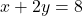, that is,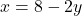Twice the first number plus the second totals 19

So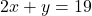Since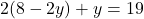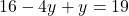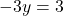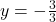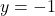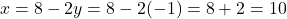The numbers are 10 and -2.